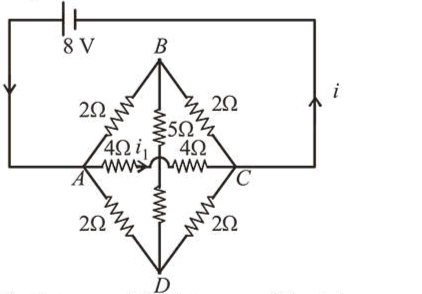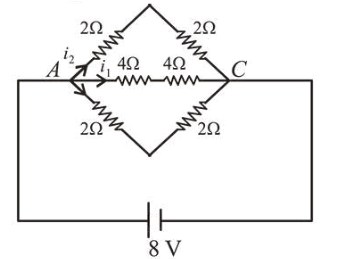# The value of current 1 flowing from A to C in the circuit diagram is :Question:

The value of current $i_{1}$ flowing from $A$ to $C$ in the circuit diagram is :1. (1) $2 \mathrm{~A}$

2. (2) $4 \mathrm{~A}$

3. (3) $1 \mathrm{~A}$

4. (4) $5 \mathrm{~A}$

Correct Option: 3,

Solution:

(3) The equivalent circuit can be drawn asVoltage across $A C=8 \mathrm{~V}$

Resistance $R_{A C}=4+4=8 \Omega$

$i_{1}=\frac{V}{R_{A C}}=\frac{8}{4+4}=1 \mathrm{Amp}$